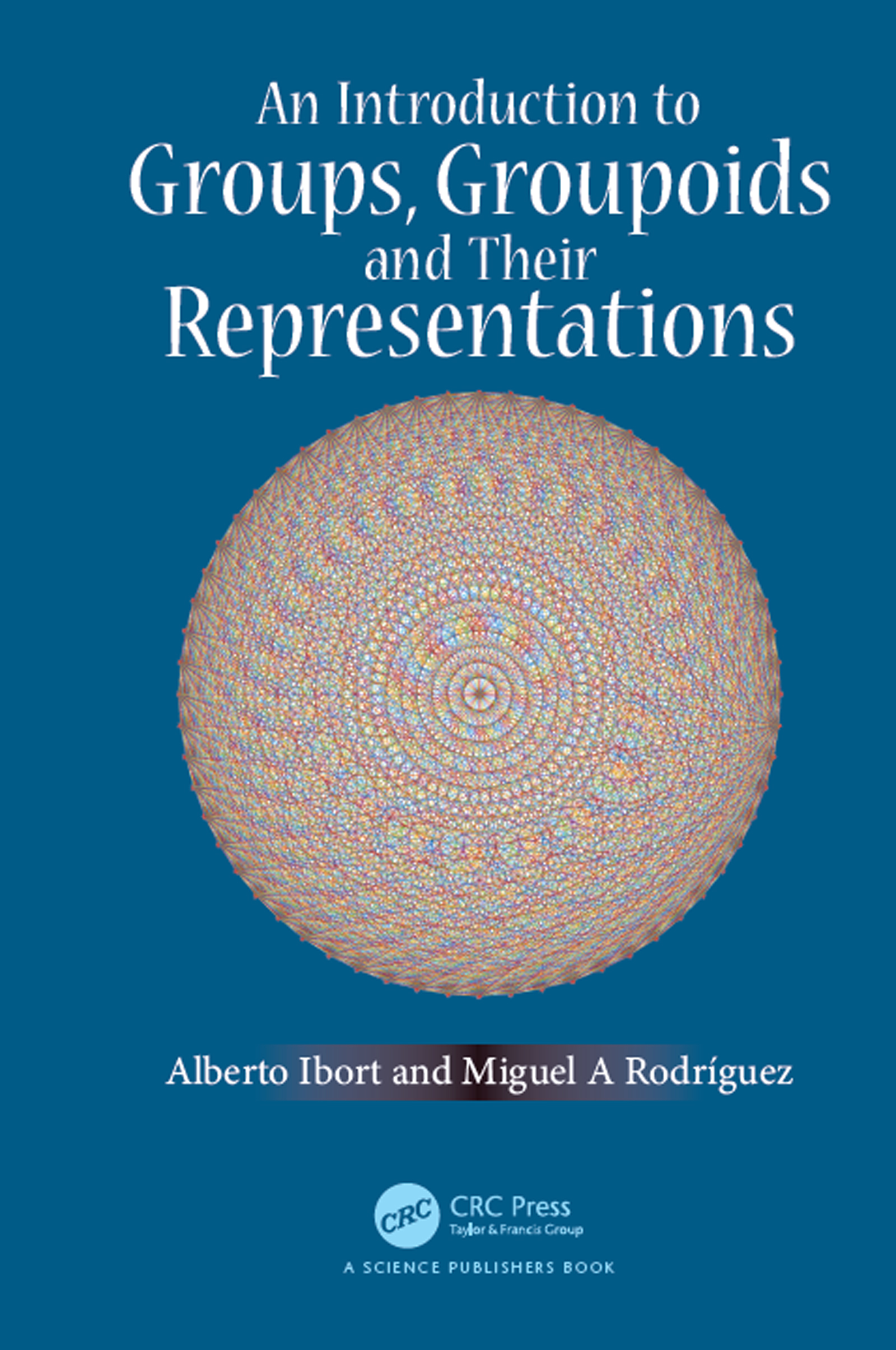An Introduction to Groups, Groupoids and Their representations

1st Edition

CRC Press

350 pages

Hardback: 9781138035867
pub: 2019-10-15
Available for pre-order
\$159.95
x

FREE Standard Shipping!

Description

This book offers an introduction to the theory of groupoids and their representations encompassing the standard theory of groups. Using a categorical language, developed from simple examples, the theory of finite groupoids is shown to knit neatly with that of groups and their structure as well as that of their representations is described. The book comprises numerous examples and applications, including well-known games and puzzles, databases and physics applications. Key concepts have been presented using only basic notions so that it can be used both by students and researchers interested in the subject.

Category theory is the natural language that is being used to develop the theory of groupoids. However, categorical presentations of mathematical subjects tend to become highly abstract very fast and out of reach of many potential users. To avoid this, foundations of the theory, starting with simple examples, have been developed and used to study the structure of finite groups and groupoids. The appropriate language and notions from category theory have been developed for students of mathematics and theoretical physics. The book presents the theory on the same level as the ordinary and elementary theories of finite groups and their representations, and provides a unified picture of the same. The structure of the algebra of finite groupoids is analysed, along with the classical theory of characters of their representations.

Unnecessary complications in the formal presentation of the subject are avoided. The book offers an introduction to the language of category theory in the concrete setting of finite sets. It also shows how this perspective provides a common ground for various problems and applications, ranging from combinatorics, the topology of graphs, structure of databases and quantum physics.

I WORKING WITH CATEGORIES AND GROUPOIDS

1. Categories: basic notions and examples

Introducing the main characters

Categories: formal definitions

A categorical definition of groupoids and groups

2. Groups

Groups, subgroups and normal subgroups: basic notions

The symmetric group

Group homomorphisms and Cayley's theorem

The alternating group

Products of groups

3. Groupoids

Groupoids: basic concepts

Puzzles and groupoids

4. Actions of groups and groupoids

Symmetries, groups and groupoids

The action groupoid

Symmetries and groupoids

Weinstein's tilings

Cayley's theorem for groupoids

5. Functors and transformations

Functors

An interlude: categories and databases

Homomorphisms of groupoids

Equivalence: Natural transformations

6. The structure of groupoids

Normal subgroupoids

Simple groupoids

The structure of groupoids: second structure theorem

Classification of groupoids up to order 20

Groupoids with Abelian isotropy group

II REPRESENTATIONS OF FINITE GROUPS AND GROUPOIDS

7. Linear representations of groups

Linear and unitary representations of groups

Irreducible representations

Unitary representations of groups

Schur's lemmas for groups

8. Characters

Orthogonality relations

Characters

Orthogonality relations of characters

Inequivalent representations and irreducibility criteria

Decomposition of the regular representation

Tensor products of representations of groups

Tables of characters

Canonical decomposition

An application in quantum mechanics: spectrum degeneracy

9. Linear representations of categories

Linear representations of categories

Properties of representations of categories

Linear representations of groupoids

10. Algebras and groupoids

Algebras

The algebra of a category

The algebra of a groupoid

Representations of Algebras

Representations of groupoids and modules

11. Semi-simplicity

Irreducible representations of algebras

Semi-simple modules

The Jordan-Holder theorem

Characterizations of semi-simplicity

The algebra of a finite groupoid is semi-simple

12. Representations of groupoids

Characters again

Operations with groupoids and representations

The left and right regular representations of a finite groupoid

Some simple examples

Discussion

III APPENDICES

A Glossary of Linear Algebra

B Generators and relations

C Schwinger Algebra

Bibliography

Index

Alberto Ibort is full professor of Applied Mathematics in the Department of Mathematics of the Universidad Carlos III of Madrid, Spain and member of the Mathematical Institute, ICMAT, Madrid, Spain. He has been visiting professor and Fulbright Scholar at the University of California at Berkeley, USA, postdoc at the Université de Paris VI, France and the Niels Bohr Institute, Denmark, and professor of Theoretical Physics at the Universidad Complutense of Madrid. His research includes several areas of Mathematics and Mathematical Physics: Functional Analysis, Differential Geometry and more recently algebraic structures on Physics and Engineering, mainly control theory.

Miguel A. Rodríguez is full professor in the Department of Theoretical Physics of Universidad Complutense of Madrid, Spain. His teaching is mainly related to courses on Mathematics applied to Physics, in particular group theory. He has been visiting professor at Université de Montréal, Canada, University of California at Los Angeles, USA, and Università di Roma Tre, Italy. His research field includes several areas of Mathematical Physics: Integrable Systems, Group Theory, and Difference Equations.